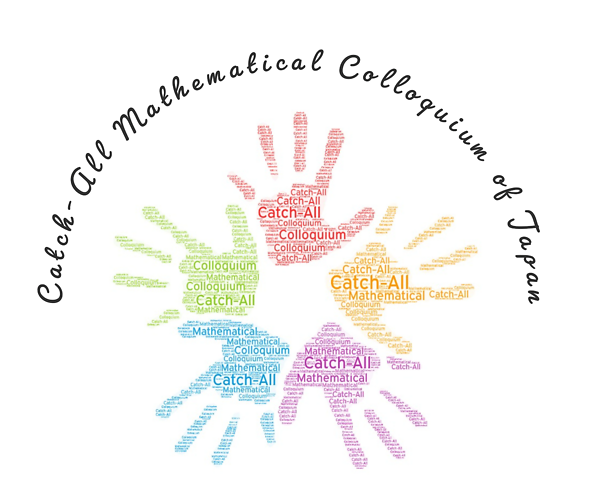# [Catch-All Mathematical Colloquium] The level-set mean curvature flow equation versus the total variation flow equation,Yoshikazu Giga (University of Tokyo )### Date

2022年5月12日 (木) 16:00 18:00

Online via Zoom

### Description

Title: The level-set mean curvature flow equation versus the total variation flow equation

Abstract: The level-set mean curvature flow equation has been introduced to track an evolving hypersurface by its mean curvature after it develops singularities. A level-set of a solution of the level-set mean curvature flow equation moves its mean curvature. The total variation flow equation is often used to remove noise from images. Although these two equations look similar, analytic properties are quite different; the former equation is a local equation while the latter is a nonlocal equation. In this talk, we compare these two equations as well as a few applications.

Discussion Theme (for the 2nd part of the event) :

How to collaborate with researchers other than mathematicians

The colloquium will be held once a month online. Each event consists of a one-hour talk on mathematics followed by a one-hour diversity panel discussion session. Please register before May 8, 5 pm. Click here to register!

All-OIST Category: